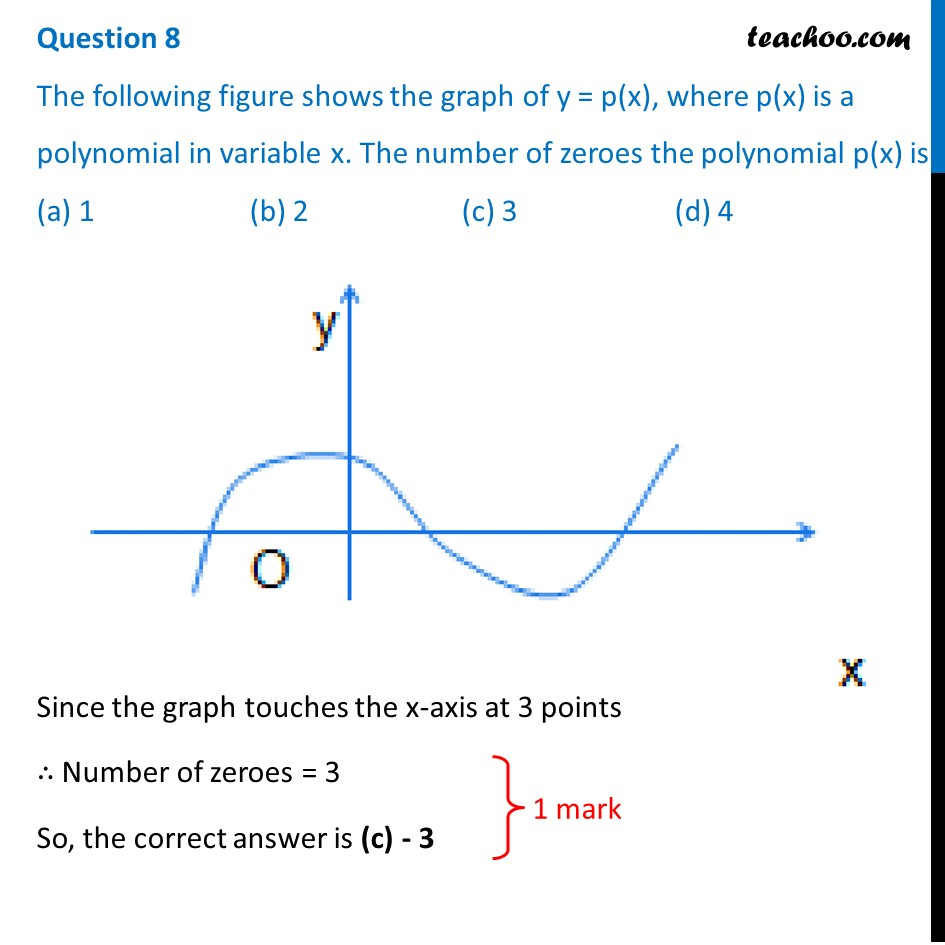## (a) 1           (b) 2            (c) 3           (d) 41. Class 10
2. Solutions of Sample Papers for Class 10 Boards
3. CBSE Class 10 Sample Paper for 2020 Boards - Maths Basic

Transcript

Question 8 The following figure shows the graph of y = p(x), where p(x) is a polynomial in variable x. The number of zeroes the polynomial p(x) is (a) 1 (b) 2 (c) 3 (d) 4 Since the graph touches the x-axis at 3 points ∴ Number of zeroes = 3 So, the correct answer is (c) - 3

CBSE Class 10 Sample Paper for 2020 Boards - Maths Basic

Class 10
Solutions of Sample Papers for Class 10 Boards